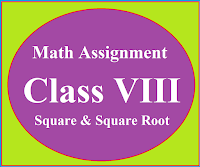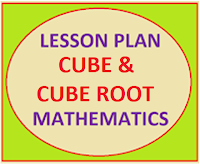## Posts

Showing posts from May, 2023

### Math Assignment Class VIII | Square & Square RootMath Assignment  Class VIII | Square & Square Root Download or Print free  assignment with answer key  for   Class  8 Squares and  Square Roots.   Important and extra questions that cover all topics of square and square root and is useful and helpful for the students. Math Assignment  Class VIII | Square & Square Root LEVEL -1

### Mind Map Mathematics, Class 10MIND MAP ACTIVITIES  FOR THE STUDENTS OF CLASS X To remember and understand the mathematical concepts easily Mind Maps are very useful. Mind maps is a fast way to revise the concepts, so these are also be called the concept maps

### Math Assignment Class VIII | Square & Square RootMath Assignment  Class VIII | Square & Square Root Download or Print free  assignment with answer key  for   Class  8 Squares and  Square Roots.   Important and extra questions that cover all topics of square and square root and is useful and helpful for the students. Math Assignment  Class VIII | Square & Square Root LEVEL -1

### Lesson Plan Math Class 8 (Ch-2) | Cube & Cube RootsE- LESSON PLAN   SUBJECT MATHEMATICS    CLASS- 8 Lesson Plan for CBSE mathematics class 8 Cube and Cube Roots, Step by step teaching strategy for mathematics teachers. Perfect lesson plan which makes the teaching learning process perfect E-LESSON PLAN MATHEMATICS CLASS-VIII CHAPTER-2 CUBE & CUBE ROOTS NAME OF THE TEACHER DINESH KUMAR CLASS VIII CHAPTER 02 SUBJECT MATHEMATICS TOPIC CUBE & CUBE ROOTS  DURATION : 10 Class Meetings PRE- REQUISITE KNOWLEDGE:- General Tables from 2 to 20 Square table from 1 to 30 Cubic table from 1 to 20 Materials: Whiteboard and markers, Calculator Worksheets with problems related to squares and square roots. Real-life examples related to cube and cube roots. Learning Objective:  To introduce the concept of cube & cube roots to students. To teach students how to calculate the cube and cube root of a number. To help students understand the practical applications of cubes and cube roots. Learning Outcomes By the end of this lesson, students will b